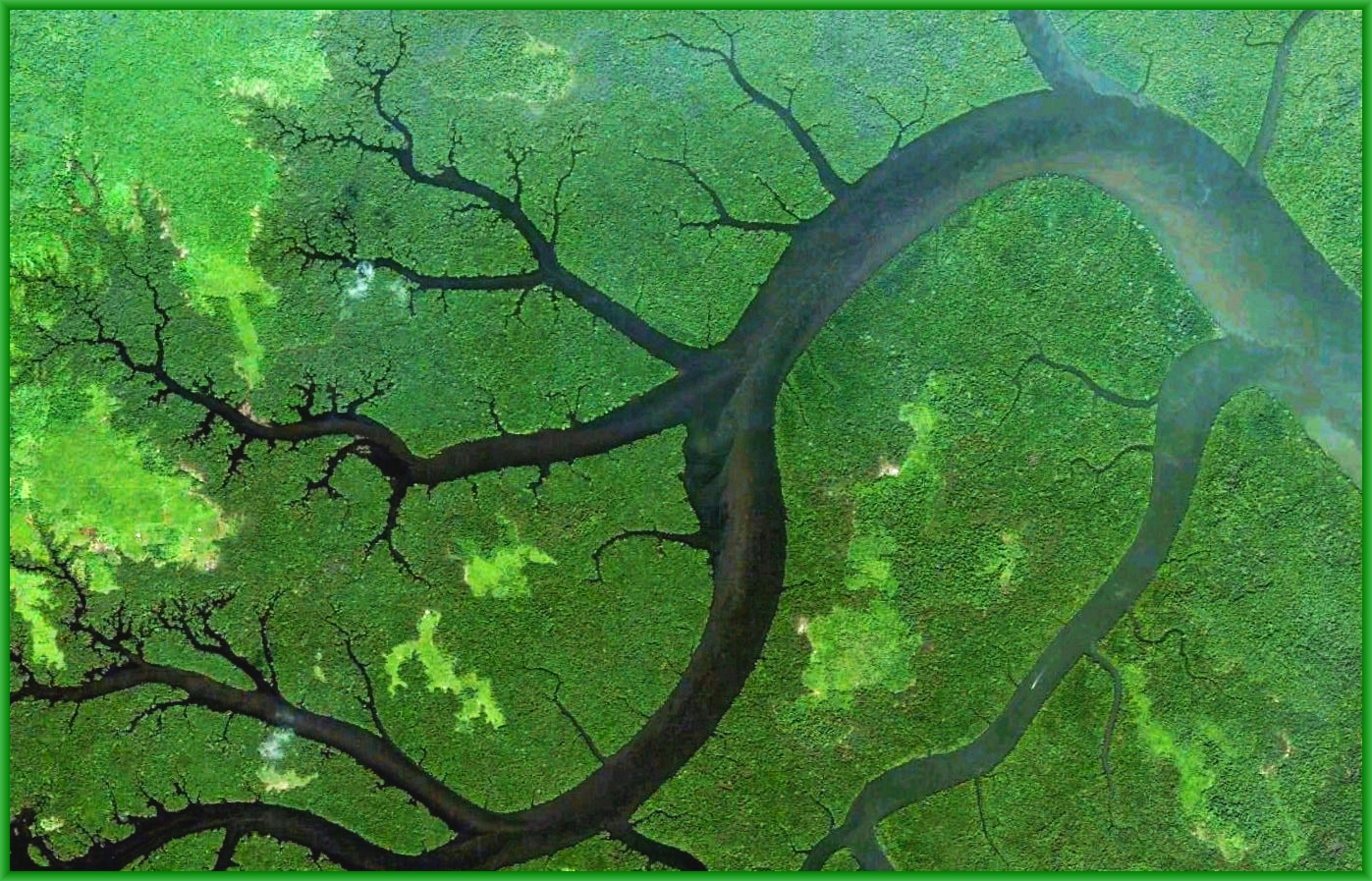## Applied Category Theory Talks at SYCO4Here are some talks on applied category theory:

#### John Baez, Props in network theory

To describe systems composed of interacting parts, scientists and engineers draw diagrams of networks: flow charts, Petri nets, electrical circuit diagrams, signal-flow graphs, chemical reaction networks, Feynman diagrams and the like. All these different diagrams fit into a common framework: the mathematics of symmetric monoidal categories. Two complementary approaches are presentations of props using generators and relations (which are more algebraic in flavor) and structured cospan categories (which are more geometrical). In this talk we focus on the former. A "prop" is a strict symmetric monoidal category whose objects are tensor powers of a single generating object. We will see that props are a flexible tool for describing many kinds of networks.

You can see the slides here. They are based on this paper:

For a gentler talk on the same theme, see:

#### Kenny Courser, Structured cospans

Category theory has played a prominent role in recent developments aimed at providing a suitable framework to better study `open networks': that is, networks with inputs and outputs. Here we introduce cospans with extra structure as a way of describing open networks.
You can see the slides here. They are based on Courser's thesis:

#### Daniel Cicala, Rewriting structured cospans

To foster the study of networks on an abstract level, we further study the formalism of "structured cospans" introduced by Baez and Courser. A structured cospan is a diagram of the form $$La \to x \leftarrow Lb$$ built from a geometric morphism with left exact left adjoint $$L \dashv R \colon \mathsf{X} \to \mathsf{A}$$. We show that this construction is functorial and results in a topos with structured cospans for objects. Additionally, structured cospans themselves are compositional. Combining these two perspectives, we define a double category of structured cospans. We then leverage adhesive categories to create a theory of rewriting for structured cospans. We generalize the result from graph rewriting stating that a graph grammar induces the same rewrite relation as its underlying graph grammar. We use this fact to prove our main result, a complete characterization of the rewriting relation for a topos $$\mathsf{X}$$ using double categories. This provides a compositional framework for rewriting systems.
You can see the slides here. They are based on Cicala's thesis:

#### Jade Master, Generalized Petri nets

We give a definition of "Q-net", a generalization of Petri nets based on a Lawvere theory Q for which many existing variants of Petri nets are special cases. This definition is functorial with respect to change in Lawvere theory and we exploit this to explore the relationships between different kinds of Q-nets. To justify our definition of Q-net, we construct a family of adjunctions for each Lawvere theory explicating the way in which Q-nets present free models of Q in $$\mathsf{Cat}$$. This gives a functorial description of the operational semantics for an arbitrary category of Q-nets. We show how this can be used to construct the semantics for Petri nets, pre-nets, integer nets, and elementary net systems.
You can see the slides here. They are based on this paper:

#### Joe Moeller, Monoidal Grothendieck construction

We lift the standard equivalence between fibrations and indexed categories to an equivalence between monoidal fibrations and monoidal indexed categories, namely weak monoidal pseudofunctors to the 2-category of categories. In doing so, we investigate the relation between this global monoidal structure where the total category is monoidal and the fibration strictly preserves the structure, and a fibrewise one where the fibres are monoidal and the reindexing functors strongly preserve the structure, first hinted by Shulman. In particular, when the domain is cocartesian monoidal, lax monoidal structures on a functor to $$\mathsf{Cat}$$ bijectively correspond to lifts of the functor to $$\mathsf{MonCat}$$. Finally, we give some indicative examples where this correspondence appears, spanning from the fundamental and family fibrations to network models and systems.
You can see the slides here. The talk is based on this paper:
and Vasilakopoulou gave a somewhat more hard-hitting talk on the same material at CT2018:

#### Christian Williams, Enriched Lawvere theories for operational semantics.

Enriched Lawvere theories are a generalization of Lawvere theories that allow there to be not merely a set of operations of each given arity, but a graph, or an object of some other category. Enriched theories can be used to equip systems with operational semantics, and maps between enriching categories can serve to translate between different forms of operational and denotational semantics. We use a definition of Lucyshyn-Wright which allows for theories to be parameterized by a monoidal subcategory of arities, and show that presentation of enriched Lawvere theories is simplified by restricting to natural number arities. We illustrate these ideas with the SKI combinator calculus, a variable-free version of the lambda calculus, presented as a graph-enriched theory.
You can see the slides here. They are based on this paper: